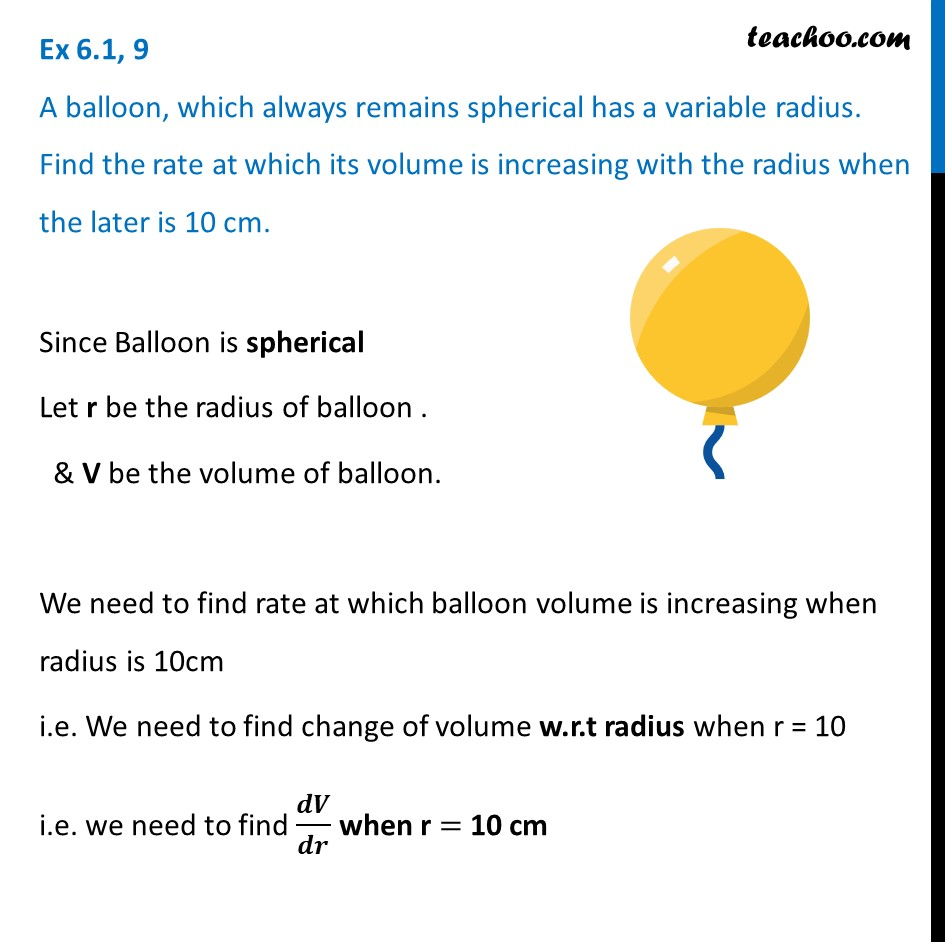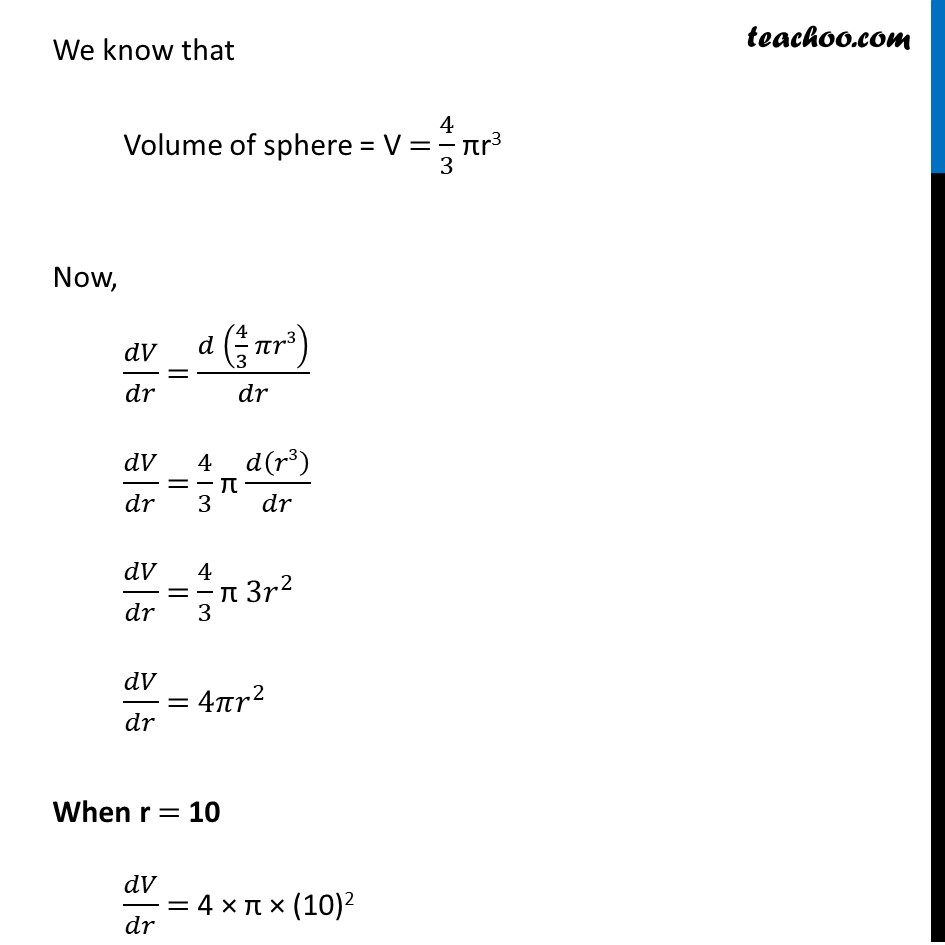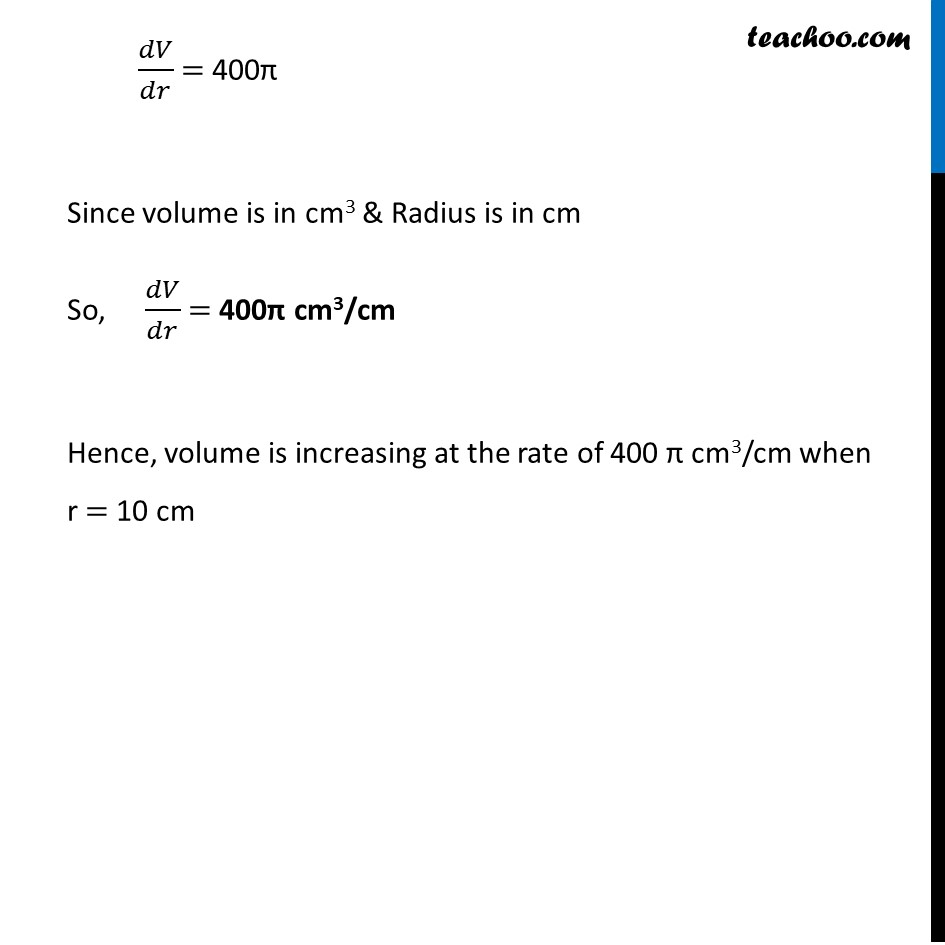1. Chapter 6 Class 12 Application of Derivatives (Term 1)
2. Serial order wise
3. Ex 6.1

Transcript

Ex 6.1, 9 A balloon, which always remains spherical has a variable radius. Find the rate at which its volume is increasing with the radius when the later is 10 cm.Since Balloon is spherical Let r be the radius of balloon . & V be the volume of balloon. We need to find rate at which balloon volume is increasing when radius is 10cm i.e. We need to find change of volume w.r.t radius when r = 10 i.e. we need to find 𝒅𝑽/𝒅𝒓 when r = 10 cm We know that Volume of sphere = V = 4/3 πr3 Now, 𝑑𝑉/𝑑𝑟 = (𝑑 (4/3 𝜋𝑟3))/𝑑𝑟 𝑑𝑉/𝑑𝑟 = 4/3 π 𝑑(𝑟3)/𝑑𝑟 𝑑𝑉/𝑑𝑟 = 4/3 π 3𝑟^2 𝑑𝑉/𝑑𝑟 = 4𝜋𝑟^2 When r = 10 𝑑𝑉/𝑑𝑟 = 4 × π × (10)2 𝑑𝑉/𝑑𝑟 = 400π Since volume is in cm3 & Radius is in cm So, 𝑑𝑉/𝑑𝑟 = 400π cm3/cm Hence, volume is increasing at the rate of 400 π cm3/cm when r = 10 cm